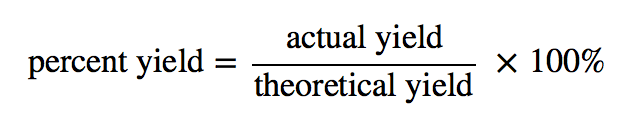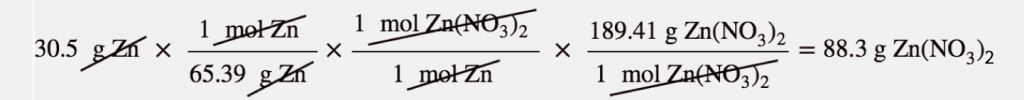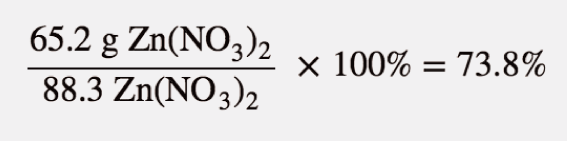# Theoretical Yield vs Percent Yield Study Guide

INTRODUCTION

Various drugs have numerous steps involved in their preparation. They also require a wide variety of expensive chemicals to support their reactions. However, chemists do a great deal of research to develop better and faster ways to make drugs evolve. Important factors like cost control include determining how much of a chemical is generated in a given reaction.

### THEORETICAL YIELD VS PERCENT YIELD

In reality, chemical reactions don’t usually go as intended on paper. Many factors result in the creation of less output than expected during the course of an experiment. Aside from spills and other laboratory failures, losses are frequently experienced due to incomplete reactions, unwanted side reactions, and so on. Chemists require a metric to determine how effective a reaction has been. The percent yield is the term for this assessment.

We determine the theoretical yield or the quantity that is hypothetically created as estimated using the balanced chemical reaction when we determine an amount of product supposing that all reactants react. Yet, in many instances, this is not the situation. During the duration of a chemical process, sometimes, much less of a product is produced. The actual yield of a reaction is this amount that is created.

Theoretical and actual yields are reported in moles or grams, respectively. A percent yield is another term that is commonly used. The percent yield is calculated by comparing the ratio of actual yield to the theoretical yield. Due to the obvious factors mentioned above, percent yields are usually less than 100%.

However, if the result obtained of the process contains impurities that cause its mass to be larger than if the product were purified, percent yields higher than 100% are feasible. When scientists create a particular chemical, they pay close attention to purifying the reaction products.

### IMPORTANCE OF PERCENTAGE YIELD

In the manufacturing of items, the percent yield is critical. Chemical producers spend a lot of time and money improving their percentage yield. When many separate processes are used to make complicated molecules, one step with a small percent yield can quickly squander a lot of reactants and cost a lot of money.

### CALCULATION OF THEORETICAL AND PERCENT YIELDSource

For example, 30.5 g of Zn reacts with nitric acid and evaporates the remaining water to obtain 65.2 g of Zn(NO₃)₂. Let us calculate the theoretical yield, the actual yield, and the percent yield.

Zn(s) + 2 HNO₃(aq) → Zn(NO₃)₂(aq) + H₂(g)

The first step is to list the quantities in the reaction:

Here, the molar mass of Zinc is 65.39 g/mol, the given mass is 30.5 also the molar mass of Zinc nitrate is 189.41 g/mol. Using the mass- mas calculation, we get that the theoretical yield of zinc nitrate is 88.3 grams.Source

The actual yield is the amount made, which, as mentioned, was 65.2 grams. To figure out the actual yield, we take the actual yield, divide it by the calculated theoretical yield and further multiply it by 100.Source

So we can state here that the reaction has achieved almost three-fourths of the possible yield.

## CONCLUSION:

• Many factors result in less output than expected during an experiment.
• We determine the theoretical yield using a balanced chemical reaction.
• The percent yield is calculated by comparing the ratio of actual yield to the theoretical yield.

### FAQs:

1. Is theoretical yield the same as percent yield?

No, the theoretical yield is an on-paper prediction, whereas percent yield is the ratio of theoretical yield and actual yield.

2. How do you find theoretical and percent yield?

In chemistry, Theoretical yield is an on-paper concept and is calculated with the assumptions of a balanced reaction with no side reactions. On the other hand, percent yield is the ratio of actual yield to theoretical yield.

3. How do you find theoretical yield given actual and percent yield?

Theoretical yield is the ratio of actual yield and percent yield multiple by hundred.

4. Why is theoretical yield different from actual yield?

Theoretical yield is different from actual yield as in reality; only a few reactions truly proceed to completion.

We hope you enjoyed studying this lesson and learned something cool about the topic of Theoretical yield vs Percent yield! Join our Discord community to get any questions you may have answered and to engage with other students just like you! Don’t forget to download our App to experience our fun VR classrooms – we promise, it makes studying much more fun! 😎

## SOURCES:

1. Theoretical Yield and Percent Yield. https://www.ck12.org/c/chemistry/theoretical-yield-and-percent-yield/lesson/Theoretical-Yield-and-Percent-Yield-CHEM/. Accessed 1 Feb 2022.
2. Yields. https://courses.lumenlearning.com/suny-introductory-chemistry/chapter/yields/. Accessed 1 Feb 2022.
]]>